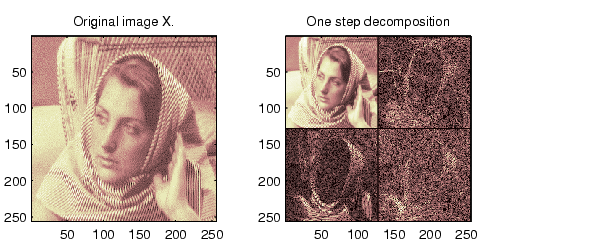Wavelet Toolboxdwt2

Single-level discrete 2-D wavelet transform

Syntax

• [cA,cH,cV,cD] = dwt2(X,'wname')
[cA,cH,cV,cD] = dwt2(X,Lo_D,Hi_D)

Description

The dwt2 command performs a single-level two-dimensional wavelet decomposition with respect to either a particular wavelet ('wname', see wfilters for more information) or particular wavelet decomposition filters (Lo_D and Hi_D) you specify.

[cA,cH,cV,cD] = dwt2(X,'wname') computes the approximation coefficients matrix cA and details coefficients matrices cH, cV, and cD (horizontal, vertical, and diagonal, respectively), obtained by wavelet decomposition of the input matrix X. The 'wname' string contains the wavelet name.

[cA,cH,cV,cD] = dwt2(X,Lo_D,Hi_D) computes the two-dimensional wavelet decomposition as above, based on wavelet decomposition filters that you specify.

• Lo_D is the decomposition low-pass filter.
• Hi_D is the decomposition high-pass filter.

Lo_D and Hi_D must be the same length.

Let sx = size(X) and lf = the length of filters; then size(cA) = size(cH) = size(cV) = size(cD) = sa where sa = ceil(sx/2), if the DWT extension mode is set to periodization. For the other extension modes, sa = floor((sx+lf-1)/2).

For information about the different Discrete Wavelet Transform extension modes, see dwtmode.

[cA,cH,cV,cD] = dwt2(...,'mode',MODE) computes the wavelet decomposition with the extension mode MODE that you specify.

MODE is a string containing the desired extension mode.

An example of valid use is

• [cA,cH,cV,cD] = dwt2(x,'db1','mode','sym');

Examples

• % The current extension mode is zero-padding (see dwtmode).

% X contains the loaded image.
% map contains the loaded colormap.
nbcol = size(map,1);

% Perform single-level decomposition
% of X using db1.
[cA1,cH1,cV1,cD1] = dwt2(X,'db1');

% Images coding.
cod_X = wcodemat(X,nbcol);
cod_cA1 = wcodemat(cA1,nbcol);
cod_cH1 = wcodemat(cH1,nbcol);
cod_cV1 = wcodemat(cV1,nbcol);
cod_cD1 = wcodemat(cD1,nbcol);
dec2d = [...
cod_cA1,     cod_cH1;     ...
cod_cV1,     cod_cD1      ...
];

% Using some plotting commands,
% the following figure is generated.Algorithm

For images, there exist an algorithm similar to the one-dimensional case for two-dimensional wavelets and scaling functions obtained from one- dimensional ones by tensorial product.

This kind of two-dimensional DWT leads to a decomposition of approximation coefficients at level j in four components: the approximation at level j + 1, and the details in three orientations (horizontal, vertical, and diagonal).

The following chart describes the basic decomposition steps for images:Note    To deal with signal-end effects involved by a convolution-based algorithm, a global variable managed by dwtmode is used. This variable defines the kind of signal extension mode used. The possible options include zero-padding (used in the previous example) and symmetric extension, which is the default mode.dwt dwtmode